## Math and Science Notations on Blackboard

Blackboard has Message Box features in its different functionalities. For example, creating annoucement, building items, creating assignments, or building test. The ribbon at the top of the Message box, right under the "Subject" box, has multiple options to choose from to include in the message. It can be regular text with different font type and size, or add audio and video files, or mathematical notations and symbols.

The mathematical notations and symbols are mostly uselful to create assignments, test questions and test options that require those notations in math and sceinces. To create those equations, you have to select the mathematical symbol option that is shown with the "fx symbol. NOTE: the WIRIS math editor does not work well, and sometimes at all, with the Microsoft Office equation editor or the Google Docs equation editor. If you do attempt to use it, please check very closely for errors.If you don't see the "fx symbol, it might be because your message box is compressed. To show all text style options, click the double drop down arrow at the to right of the message box.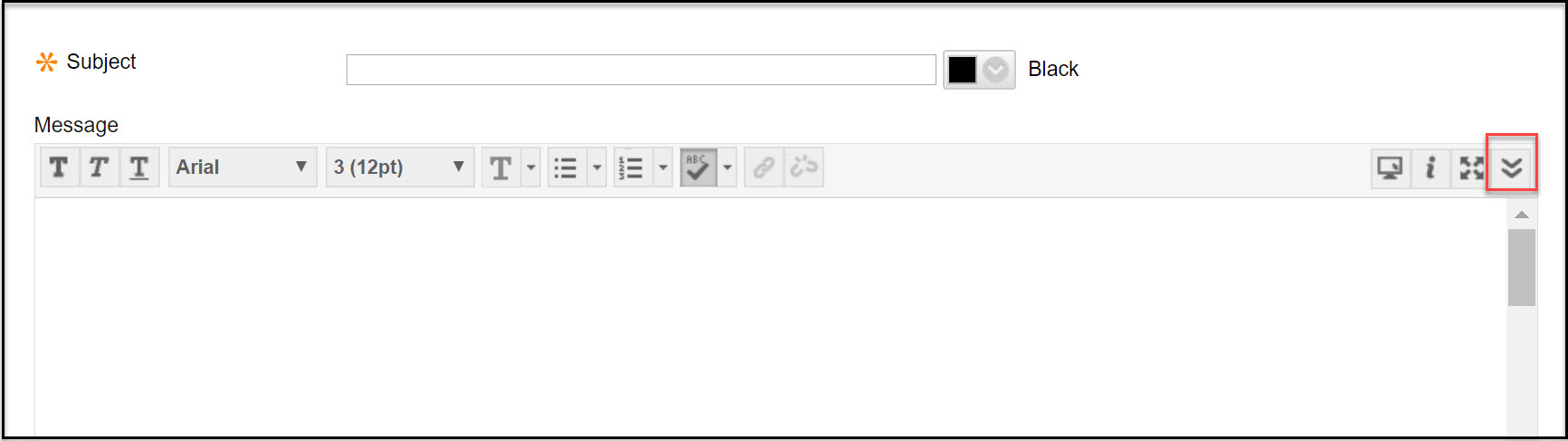Clicking the math equation option gives you a new window to write your mathematical equations on.After you write your equation, you click "Accept" to add the equation to the message, question item, or answer option you are writing, or you click "Cancel" to disregard it.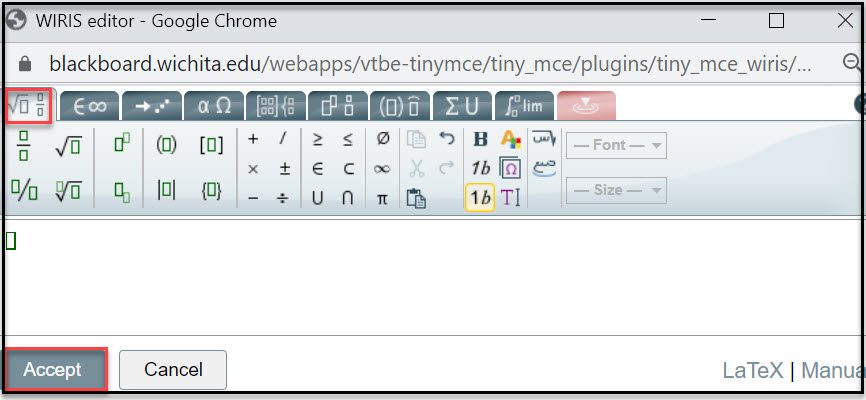If the symbol you want to include in different from what you see in the general symbols options, you might need to click on the other options that are on the top raw which will give you many more symbol options in that domain. For example, arrows, Greek symbols, matrices, and more.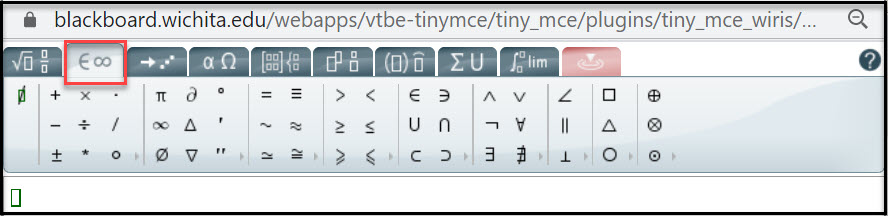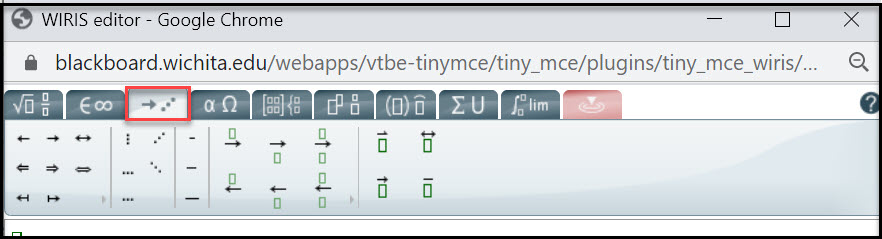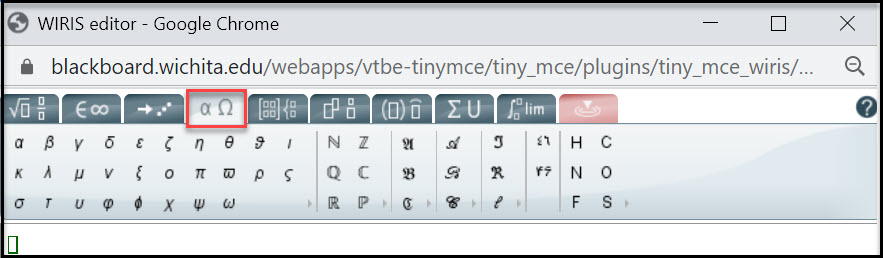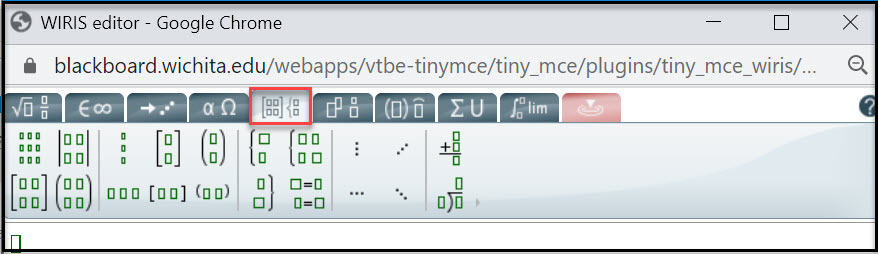There are more options than the ones seen in the above images. Hoover over each domain option on the top row and see what other options are available to make your equation complete.

If you are creating mathematical question, the question item can include formula, text, equations, and anything you want to include in it. See the example below: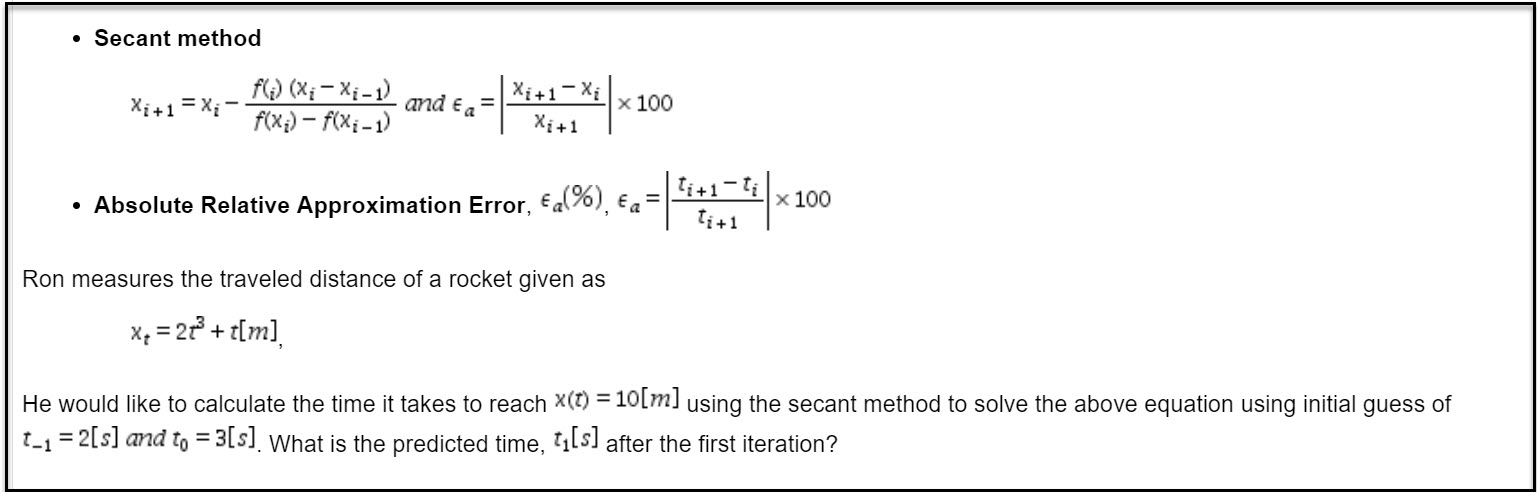If you create a multiple choice item using mathematical notations, and if the answer options also need to use those notations, you can create the eqautions same way as you did for the question item.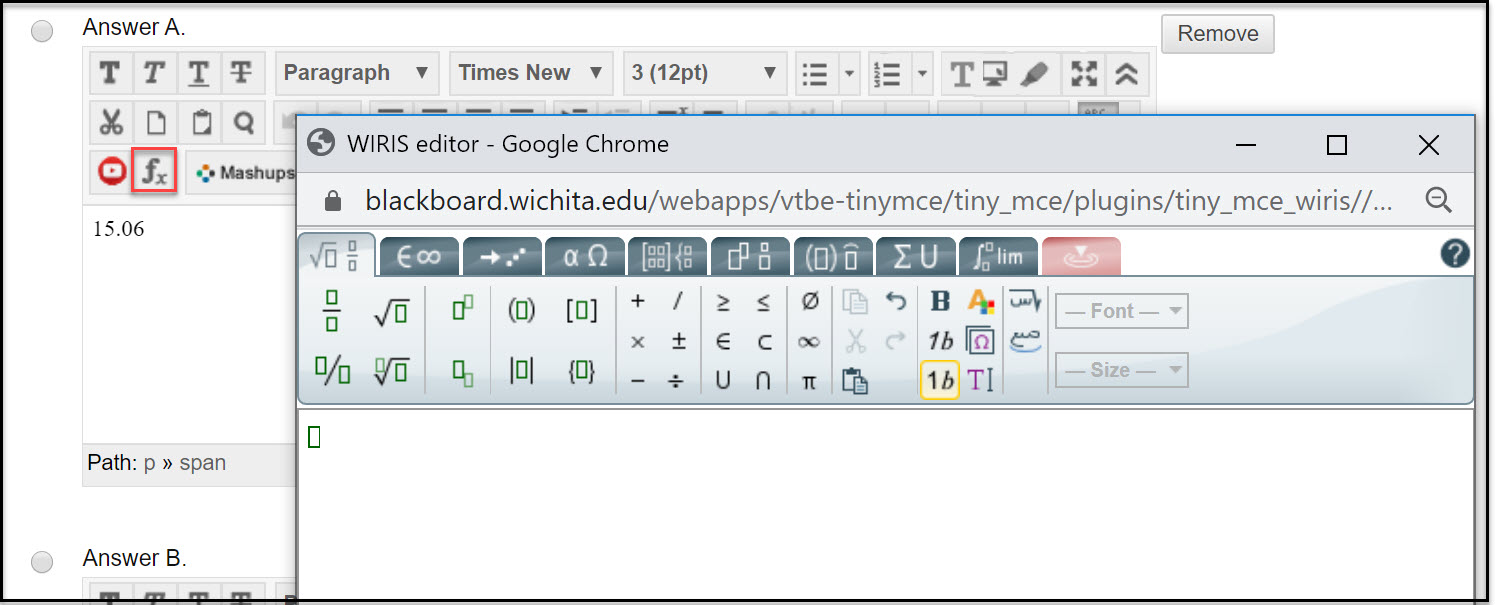For science subjects that use a different subject related symbols, you can use a software, or applications that are used in the descipline and you can copy the formula from the software or application and paste it on blackboard.

## Creating Calculated Formula Questions

A Calculated Formula question allows instructors to create questions with varying numerical values based on an instructor-determined formula. To create a calculated formula question, open the test canvas and click on Create Question, and select Calculated Formula from the menu. When the question page opens, add question text that includes variables in square brackets [x] corresponding to variables in the formula that will be used to calculate the answer to this question.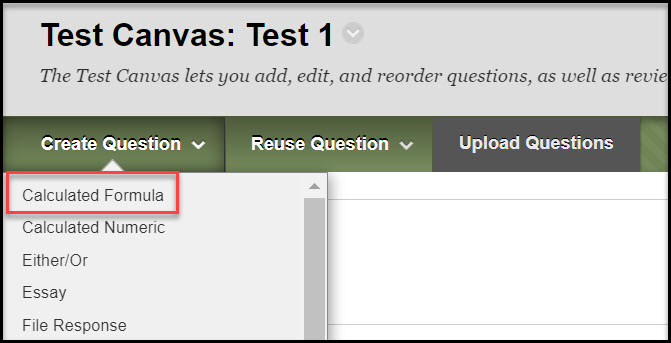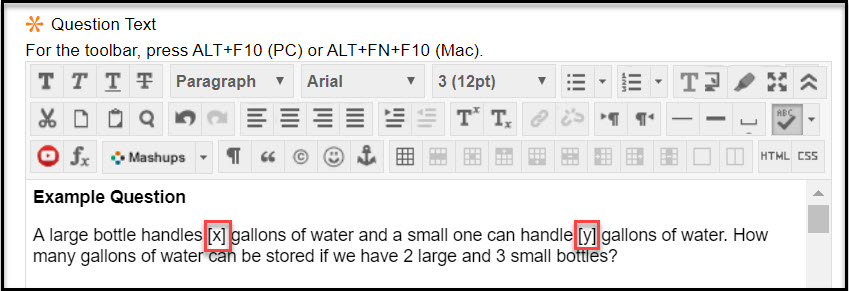Answers are not part of the formula because answer sets are automatically calculated. Variables must consist of letters, but "pi" and "e" are reserved and cannot be used as variables. The answer formula for the example question in the image above will look as seen below.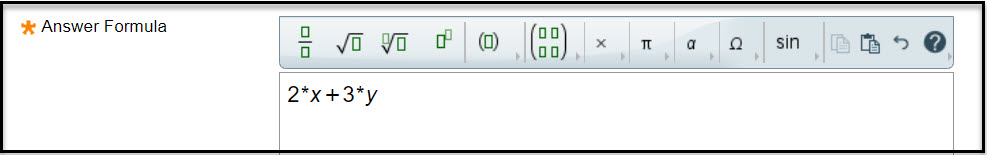After intering the question and answer formula, define the correct answer range, plus or minus a numeric or a percentage variation from the exact answer. If the correct answer must be exact, the range should be 0. If partial credit is allowed, define the broader range for partial credit and the percentage of the total points that will be given if the answer is within the partial credit range. For example, units can be required as part of the answer, and a student may lose points if the units are missing or are  incorrect. After completing the fields on this page to calculate answer sets and provide response feedback, click Next.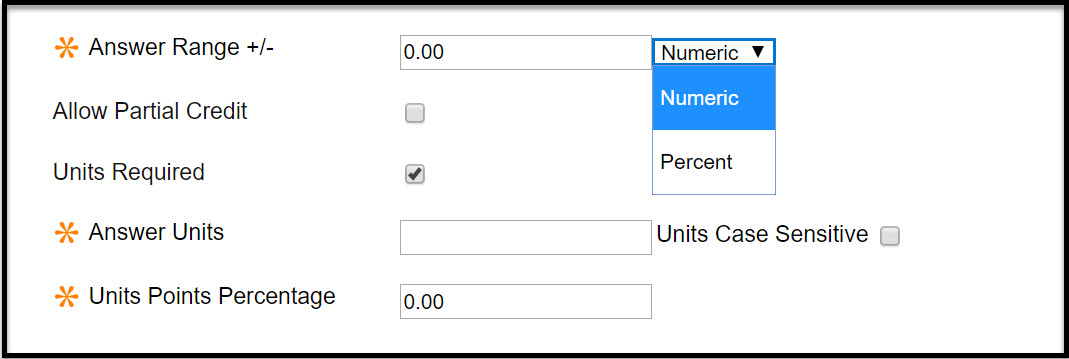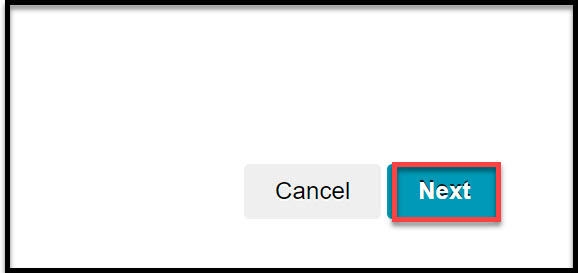The next step is defining your variables and answer sets. Variables can be expressed in either normal or exponential notation. For example, -0.0001 can be written as 1.0e-4 or as 1.0E-4 without spaces. Studennts' answeres can also be expressed in standard or exponential notation.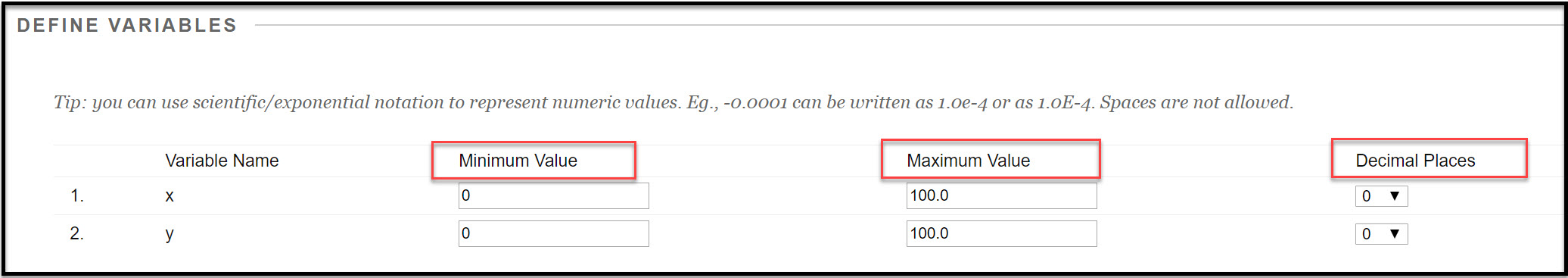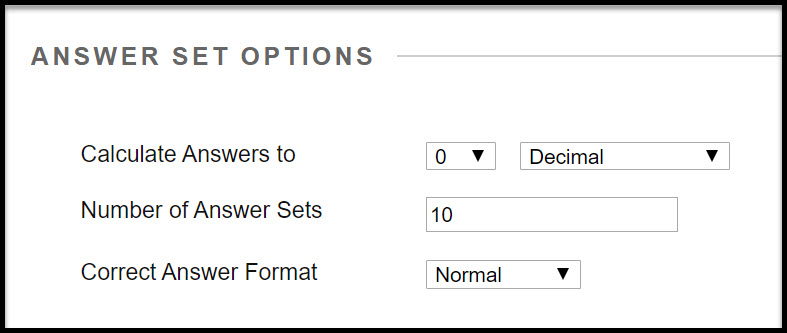Specify the the minimum and maximum values for all your variables, and at the answer set option, specify the number of decimal places or significant digits to calculate the answer to and the possible number of answer sets to generate to students. You can make every stduent get a different value for each variable by specifying the number of answer sets the same amount as the number of students taking the test.
You can then c
lick Calculate to generate new answer sets , or Next to go to the next page to adjusting the number of answer sets as required but without recalculating existing answer sets if answer sets have already been generated. Click Back to go back to the previous page. Click Cancel to quit.

You will now be able to view the system generated answer sets. You can change any of the variable values by inputing new values, To remove an answer set, click the Remove button. If you change any variable values, click the Calculate button to calculate new answer values.

Once you are done creating your question, you can submit and close or submit and create a new calculated formula question.